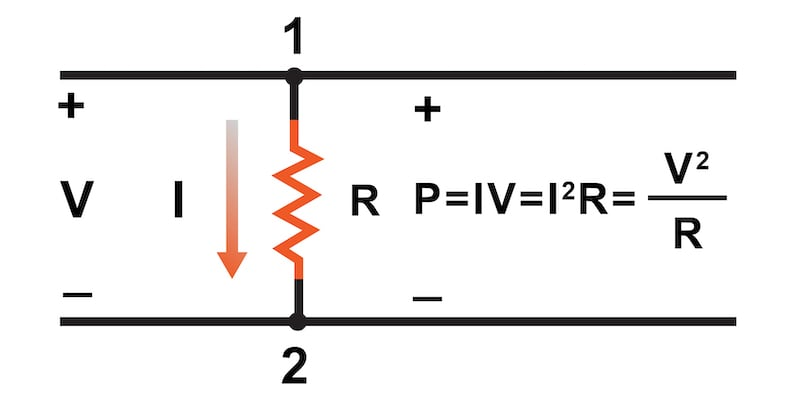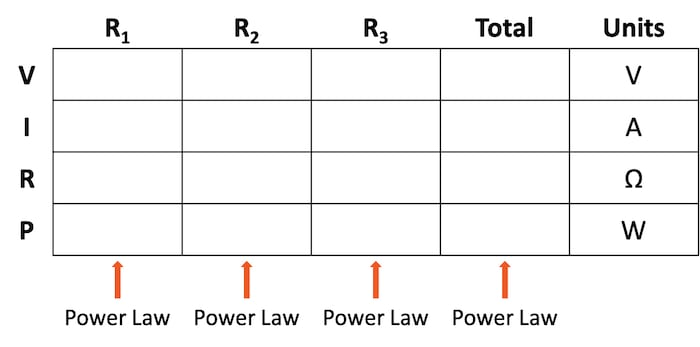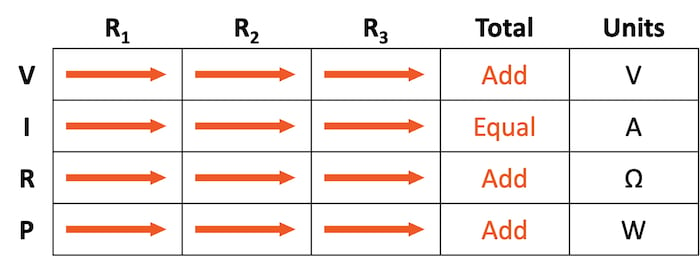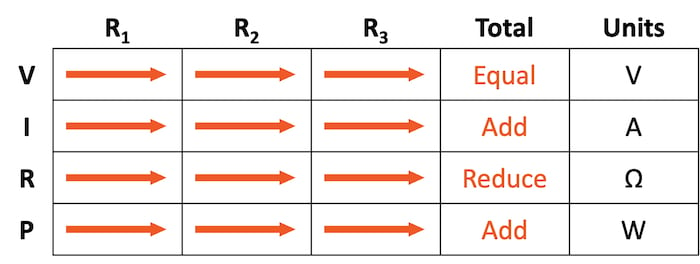Vol. Direct Current (DC)
Chapter 5 Series And Parallel Circuits

# How to Calculate Power in a Series and Parallel Circuit### What is Electrical Power and How Can You Calculate it in Series and Parallel Circuits?

Electrical power measures the rate of work represented in electrical circuits by the symbol “P” and the units of Watts (W). The total circuit power is additive for series, parallel, or any combination of series and parallel components.

When calculating the power dissipation of resistive components, we can use any one of the three Ohm’s law power equations if given any two of the voltage (V), current (I), and resistance (R):

$$P = IV = I^2R = \frac{V^2}{R}$$

### Calculating Power Using the Table Method

These electric power calculations can be easily managed using the table method by adding another row below the voltages, currents, and resistances, as shown in Table 1.

##### Table 1. Table method with power included.Power for any particular table column can be found using the appropriate Ohm’s power law equation.

### Power in Series and Parallel Circuits

Power is a measure of the rate of work. Per the physics law of conservation of energy, the power dissipated in the circuit must equal the total power applied by the source(s). Therefore, an interesting rule for total circuit power versus individual component power is that it is additive for any circuit configuration: series (Table 2), parallel (Table 3), or any combination of series and parallel.

##### Table 2. Table method for series circuits—power is additive.##### Table 3. Table method for parallel circuits—power is additive.If you need a refresher or skipped the pages on series circuits and parallel circuits, you can find them here:

### Review of Power for Series and Parallel Circuits:

• Electrical power is the measure of work
• Power is represented by the symbol “P”
• The unit of power is the Watt (W)
• Power is additive in any configuration of a resistive circuit—series, parallel, or series-parallel
• Ptotal = P1 + P2 + . . . Pn

### Related Content

Calculators:

Worksheets

Video Tutorials and Lectures: